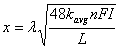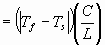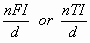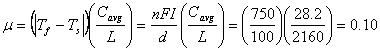# Modified Berggren Formula

The modified Berggren formula was developed in the early 1950s to address the shortcomings of the Stefan formula.  The modified Berggren formula assumes that the soil is a semi-infinite mass with uniform properties and existing initially at a uniform temperature (Ti).  It is further assumed that surface temperature is suddenly changed from Ti to Ts (below freezing). The modified Berggren formula is simply the Stefan formula corrected for the effects of temperature changes in the soil mass:where: x equals depth of freeze or thaw, (ft)) λ equals dimensionless coefficient which takes into consideration the effect of temperature changes in the soil mass (i.e., a fudge factor). Corrects the Stefan formula for the neglected effects of volumetric heats (accounts for "sensible heat" changes) kavg equals thermal conductivity of soil, average of frozen and unfrozen (BTU/hr • ft • °F) n equals conversion factor for air freezing (or thawing) index to surface freezing (or thawing) index FI equals air freezing index (°F • days) TI equals air thawing index (°F • days) L equals latent heat (BTU/ft3)

## Determination of λ

l can be determined by chart (Figure 1) based on inputs of a (thermal ratio) and m (fusion parameter).

 λ equals f (FI (or TI), mean annual air or ground temperature, thermal properties of soil) equals f(m,a) and can be read from Figure 1 μ equals fusion parameterC equals average volumetric heat capacity of a soil (BTU/ft3 • °F) L equals latent heat (BTU/ft3) equals surface freezing (or thawing) index, nFI (or nTI) divided by length of freezing (or thawing) season. Represents temperature differential between average surface temperature and 32 °F taken over the entire freeze (or thaw) season. equalsd equals length of freezing or thawing duration. For example, if the winter freezing season is December through February, then the duration of freezing (d) equals about 90 days. Tf equals 32 °F Ts equals average surface temperature for the freezing (or thawing) period α equals thermal ratioT equals average annual air or ground temperature |T - Tf| equals represents the amount that the mean annual temperature exceeds (or is less than) the freezing point of the soil moisture (assumed to be 32 °F).

## Modified Berggren Formula Example

Determine the depth of frost penetration into a homogeneous sandy silt for the following conditions:

Given:

• Mean annual temperature = 48 °F
• Surface freezing index = nFI = 750 °F • days
• Duration of freezing season = d = 100 days
• Soil properties:
• Dry density = gd = 100 lb/ft3
• Water content = w = 15%

Solution

• Calculate soil thermal properties

Volumetric latent heat of fusion:
L = (144 BTU/lb) (100 lb/ft3) (15/100) = 2160 BTU/ft3

Average volumetric specific heat:
Cavg = 100 (lb/ft3) (0.15) = 28.2 BTU/ft3 • °F

Average thermal conductivity:
kf@ 0.80 BTU/hr • ft • °F
ku@ 0.72 BTU/hr • ft • °F

Therefore, kavg = 0.76 BTU/hr • ft • °F

• Calculate λ (recall λ = f(α, μ))From the chart, λ= 0.74

• Calculate depth of freezing